Peak torque comparison between axial and radial flux machines

Welcome back! Last time, we dived into the exciting world of axial-flux machiness. However, the dive was quite shallow, as we only covered their structure and some basic principles. But fear not, this time we are going to do some performance comparisons!

Indeed, we are going to estimate the maximum torque density of a machine of given size. Even better, we are going to compare axial-flux machines to radial-flux ones, to see which is better in which case!

Sidenote: This is a blog. Meaning, I didn’t triple-check my results. (Although triple-secing them would make ’em much more enjoyable…I digress.) I hardly even single-checked them. So, for the love of everything’s that good, don’t make business or career decisions based on this.

Sidenote #2: You can now check the codes yourself at Github. The file plots_1a.m does the optimization and makes the plots, and I’m sure you can find your way to the rest of the functions.

Here’s how we’re gonna do it.

## Rules

We are gonna fix the total motor diameter to 20 centimeters. That means the core diameter for radial-flux machines, and core plus end-winding for axial. Then, we’re gonna change the length (again the total value including the end-winding) to see how the torque-per-volume changes.

Surface-PM topology is used, and the airgap is fixed to 0.5 mm. The remanence of the PMs is 1.35 T, and inside the core we’ll accept 1.9 in the stator teeth and rotor yoke, and 1.7 T in the stator yoke. The number of poles and slots will be varied, but always as a multiple of the 10/12. The winding factor is assumed to be 0.95.

To reiterate, we only consider maximum torque here. Steady-state has been analysed ad nauseum, plus it requires careful thermal analysis to get good results.

In the analysis to follow, a few different current densities will be used. These are high and probably would not work in continuous use without heavy-duty cooling, but still realistic for short peak loads.

Furthermore, the maximum electric loading (linear current density) will be bound from above. Meaning, the total current (Ampere-turns) per air-gap circumference must stay below a certain value.

This is for two reasons.

### Why limit K?

First of all, ridiculously high values can demagnetize parts of the PMs. For instance, Neodymium magnets might start to get demagnetized at 600 kA/m field strength. And that’s the actual field strength inside the magnet, while the surface current density represents the average field over the slot pitch. In practice, local values can be much higher near the slot openings.

Secondly, high values increase the slot leakage flux. After all, both quantities (electric loading and leakage flux) are proportional to the same value – total Ampere -turns per slot. The leakage flux can then oversaturate the stator teeth and/or yoke. This increases the iron losses and starts to weaken the torque-per-current ratio.

This type of saturation effect is a pain to take into account in simple models, even more so in axial-flux machines where the effect can be the most prominent at the inner diameter (as the teeth are the narrowest over there). Indeed, finite element analysis would be required for precise analysis. To keep things simple – this is a blog, not a scientific journal or even a white paper – the electric loading is limited instead, and detailed saturation analysis is left to the reader.

Next, let’s take a look at the calculation models.

## Calculation models

As we are only interested in maximum torque density, we are not gonna spend time on fine-tuning rated load performance or efficiency.

Torque is all that counts.

In both topogies, we are gonna compute it with the Lorentz force approach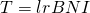.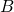is the airgap flux density while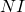is the slot mmf. That, we obtain from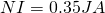,

where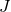is the fixed current density and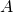is the slot area.

The airgap flux density, on the other hand, can easily be computed from the PM height and remanence, and the airgap length.

Note: rms values for both quantities, folks.

After the torque, the only remaining step is then to calculate the machine volume to obtain the volumetric torque density.

Now, let’s take a little closer look on how the calculations differ between the two machine topologies.

### Axial-flux calculation model

The free variables include:
– slot width
– PM height
– inner diameter

For axial-flux machines, slots are assumed rectangular so that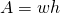.

Width times height, in other words. The moment arm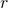is then equal to the average of the inner and outer radii of the stator core.

To compute the volume, the end-winding thickness is assumed equal to half the slot width, reflecting an ideal two-layer winding*. The EW thickness directly gives us the outer diameter, too, after being subtracted from the total outer diameter.

*quite an optimistic assumption.

Join the discussion on the Electric Motor Design group on Facebook.

The rotor yoke thickness is selected directly based on the maximum allowed flux density. In other words, the yoke is just thick enough to carry half of the flux of one pole, computed at the outermost periphery of the airgap. Finally, the stator core length is defined so that the total length is maintained at the specified value. The maximum linear current density is also checked, evaluated at the inner radius.

In this case, the free variables include
– magnet height
– tooth width
– slot height

Now, the moment armof the torque is simply the airgap radius. Uniform-width teeth are assumed, sois obtained by subtracting the tooth area from the slot-pitch sector area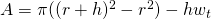.

The stator yoke height is not a free variable, but obtained directly from the other dimensions. The yoke flux density must stay below the permitted limit, of course, which sets a nonlinear constraint on the variables.

The end-winding thickness is then again assumed to be equal to half of the slot bottom width. Finally, the length of the core is defined so that the maximum allowed total length is fully used.

## Optimization

For each configuration – total length, current density, number of poles/slots, and maximum allowed linear current density – the free dimensions are then optimized to maximize the torque.

Since I lost access to Matlab’s finer optimization function after going sellsword, the optimization was performed using a simple differential evolution algorithm. It’s an easy one to code, even by looking at the pseudocode on it’s Wikipedia article alone, and often yields the global optimum to boot with. What is doesn’t do is yield the 99.9999999 % correct solution very fast, but here we’re anyways only concerned on getting in the 95% neighbourhood.

## Results

Finally, let’s look at some results. The following three pictures each represent how the maximum torque of the two topologies changes as the total length is increased from 3 cm to 10 cm. The maximum linear current density is set to 50 kA/m, 100 kA/m, and 200 kA/m, respectively.

Furthermore, each picture is divided into three subplots, organized side-by-side. Each subplot corresponds to a different current density ranging from 15 to 60 Amperes per square-millimeter.

Finally, each subplot has a total of six curves. The colors correspond to 10/12, 20/24, and 40/48 slot-pole-combinations, while the topology is indicated by the line style.

Solid lines for radial-flux motors, dashed for axial.

### So, what can we see?

Unsurprisingly, axial-flux motors seem to generally beat radial-flux ones at low total lengths. They are essentially disk-shaped, after all.

Beyond that, however, things get less straightforward. For instance, the performance gap between axial and radial flux (in favour of axial) seems to be the largest at high current densities, with a limited surface current density.

By contrast, at high electric loadings, radial-flux machines seem to perform comparably well even at short total lengths.

And naturally, radial-flux motors start to strongly dominate when the motor size is increased.

### Interpretation

So, why does this happen?

Well, that’s one problem with black-box optimization – the answer is rarely immediately clear.

Nevertheless, perhaps the most important factor is this. You remember, for a fixed electric loading, the torque capacity of a motor is more or less proportional to the airgap area times the moment arm.

Now, for an axial-flux machine with an outer diameter of 20 cm, this area is not very high. After all, the inner diameter is usually around 50 % of the outer core diameter or even more, to leave space for the winding inside the inner bore. (And the core diameter is less than 20 cm, thanks to the end-winding.) Furthermore, the average moment arm is of course the mean of the outer and inner diameters.

By contrast, a radial-flux motor with a high number of poles can have an airgap diameter near 15 cm – which directly translates to the moment arm, too. One does not need to do many calculations to see that the core length at which the math starts to favour the radial-flux topology is not that much.

Obviously, the effects of flux fringing and similar are completely and thoroughly ignored here. In practice, 3D effects like this can really start to hit the torque capacity of radial-flux motors at low core lengths. Easily in the high-teens as far as percentages go, if not more.

## Conclusion

The maximum torque capacity of axial- and radial-flux electric motors was compared with simple analytical models. Unsurprisingly, the axial topology rules at short machine lengths. However, the effect is not as pronounced as one might expect. Furthermore, really short radial-flux motors might be a good choice in some scenarios, too.

The results are a little surprising, yes, but in line with some prior research (this and this, for example).

Check out EMDtool - Electric Motor Design toolbox for Matlab.

Need help with electric motor design or design software? Let's get in touch - satisfaction guaranteed!Axial-Flux Machines – Part 2